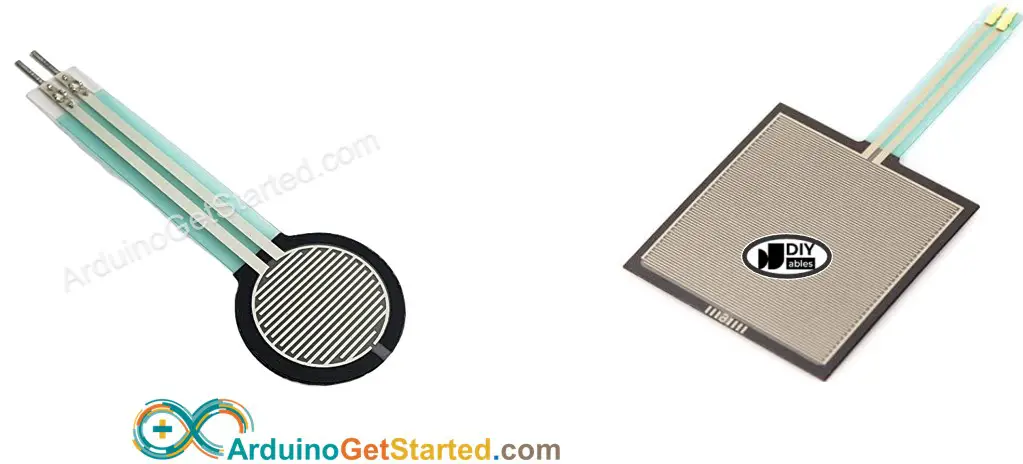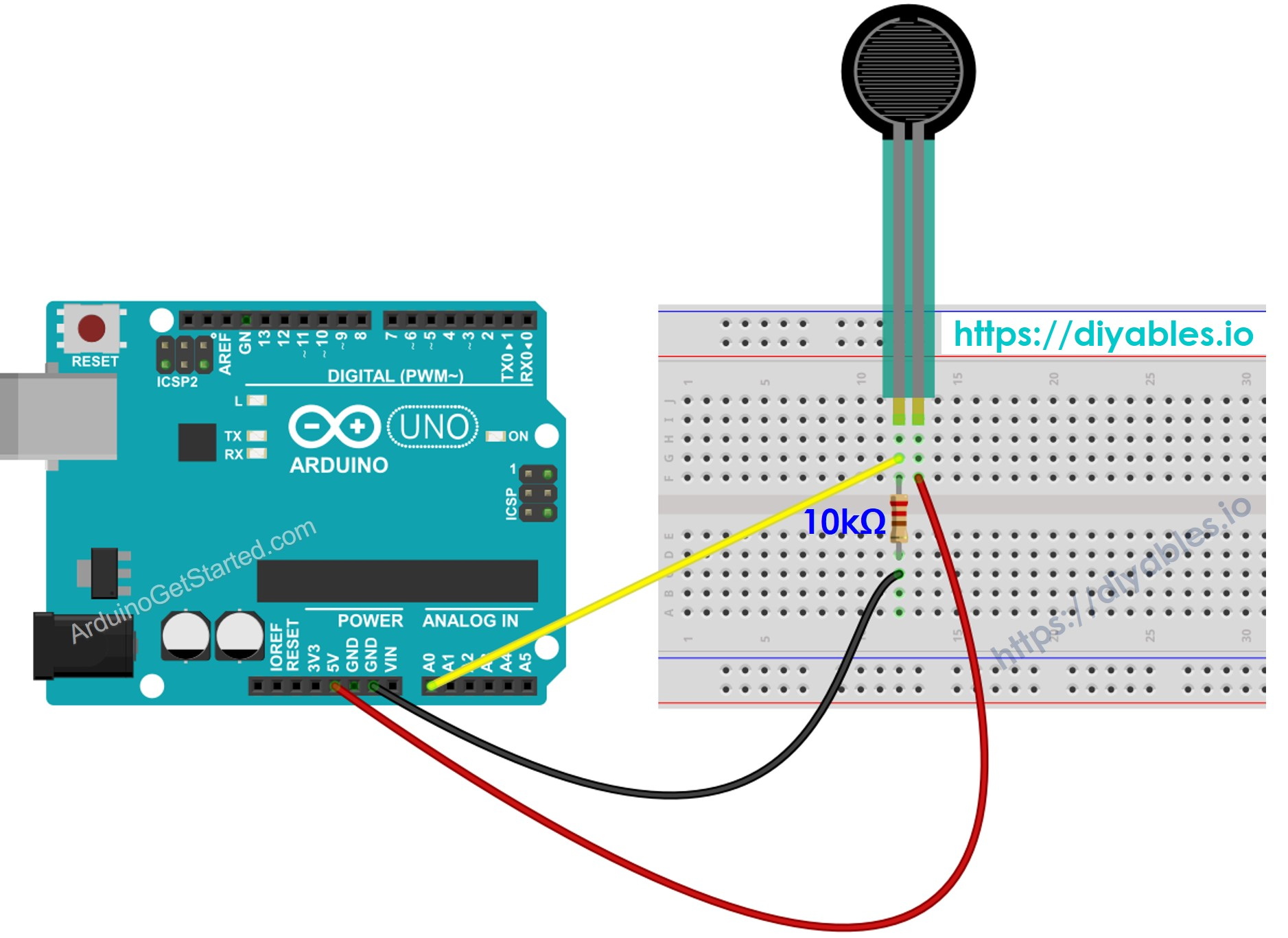# Arduino - Force Sensor

In this tutorial, we are going to learn:

• How force sensor works
• How to connect the force sensor to Arduino
• How to program Arduino to read the state from the force sensor
• How to use the force sensor with Arduino

## Hardware Required

Or you can buy the following sensor kit:

Please note: These are affiliate links. If you buy the components through these links, We may get a commission at no extra cost to you. We appreciate it.The force sensor is also known as force sensing resistor, force sensitive resistor, or just FSR. The force sensor is basically a resistor that changes its resistive value depending on how much it has been pressed. The force sensor is:

• Low-cost and easy-to-use.
• Good at detecting physical pressure, squeeze.
• Not good at finding how many pounds of weight they have on them.

The force sensor is used in electronic drums, mobile phones, handheld gaming devices and many more portable electronics.

### Pinout

A force sensor has two pins. Since it is a kind of resistor, we do NOT need to distinguish these pins. They are symmetric.

### How It Works

The force sensor is basically a resistor that changes its resistance depending on how much it has been pressed. The harder you press on the sensor, the lower the resistance between the two terminals will be.

## Wiring DiagramThis image is created using Fritzing. Click to enlarge image

## How To Program For Force Sensor

Arduino Uno's pin A0 to A5 can work as the analog input. The analog input pin converts the voltage (between 0v and VCC) into integer values (between 0 and 1023), called ADC value or analog value.

By connecting a pin of the force sensor to an analog input pin, we can read the analog value from the pin by using analogRead() function, and then we can know how much it has been pressed.

## Arduino Code

/* * Created by ArduinoGetStarted.com * * This example code is in the public domain * * Tutorial page: https://arduinogetstarted.com/tutorials/arduino-force-sensor */ #define FORCE_SENSOR_PIN A0 // the FSR and 10K pulldown are connected to A0 void setup() { Serial.begin(9600); } void loop() { int analogReading = analogRead(FORCE_SENSOR_PIN); Serial.print("Force sensor reading = "); Serial.print(analogReading); // print the raw analog reading if (analogReading < 10) // from 0 to 9 Serial.println(" -> no pressure"); else if (analogReading < 200) // from 10 to 199 Serial.println(" -> light touch"); else if (analogReading < 500) // from 200 to 499 Serial.println(" -> light squeeze"); else if (analogReading < 800) // from 500 to 799 Serial.println(" -> medium squeeze"); else // from 800 to 1023 Serial.println(" -> big squeeze"); delay(1000); }

#### Quick Steps

• Copy the above code and open with Arduino IDE
• Click Upload button on Arduino IDE to upload code to Arduino
• Press the force sensor
• See the result on Serial Monitor.
COM6
Autoscroll Show timestamp
Clear output
9600 baud
Newline

## Video Tutorial

We are considering to make the video tutorials. If you think the video tutorials are essential, please subscribe to our YouTube channel to give us motivation for making the videos.